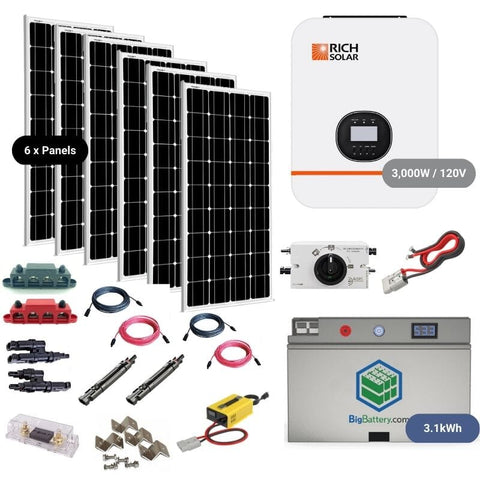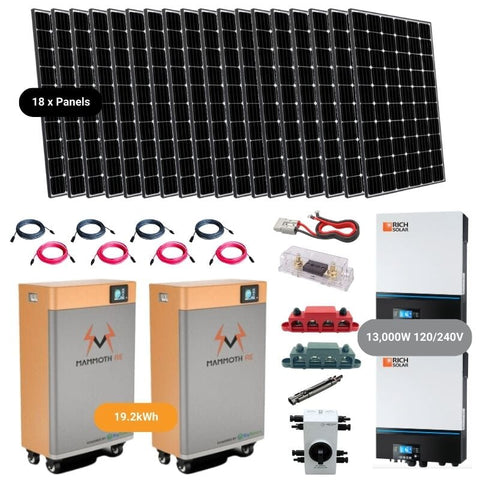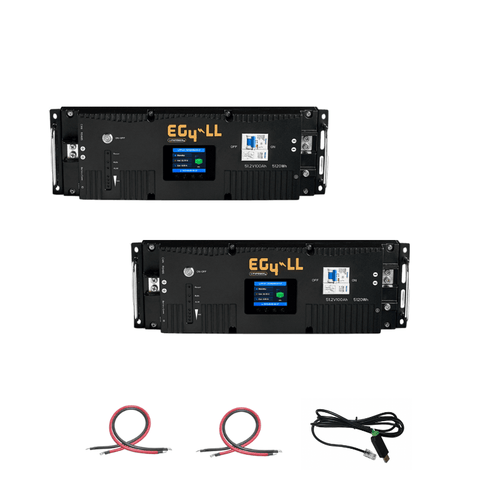Ah to kWh Calculator - The How & Why - ShopSolar.com

# Ah to kWh Calculator

The world of solar and electrical power has hundreds of different calculators that help us with conversion and overall problem-solving.

We’re going to go over how to use an ah to kWh calculator, breaking down what’s needed and how the conversion works.

Before we begin looking at our Ah to kWh calculator, we need to dissect it and look at the variables as individuals.

## What Is an Amp Hour?

When you’re new to the solar world, you’ll hear about all sorts of units of power and different conversions or calculators.For example, you may come across a calculator that can convert cold-cranking amps to amp hours but not know what either of these measurements is used for.

Today, we’re going to focus on the latter to get a better understanding of it

Amp hours are a way to measure the amount of time that a battery stays powered.

All batteries have a specific capacity: the maximum amount of energy that can be used under normal circumstances. This capacity is typically measured in amp hours.

Let’s look at an example to get a better understanding.

### Example

If you have a battery that has a capacity of 1 Ah and it requires a current draw of exactly 1 A, the battery can run an appliance for a single hour.

If we take this hour and divide it by 10, it tells us that every 6 minutes, the battery depletes by 10%.

You can use an online amp hour calculator to plug in more complex numbers but the basic premise remains.

If you don’t know where to start, use our house amp calculator. This will tell you what you need and help you decide on the size of battery you need to get going.Now that we know all about amp hours, what about kilowatt hours?

## What Are Kilowatt Hours?

Aside from the calculators used to convert MWh to kWh, the most common place you’ll see this unit is at the bottom of your monthly power bill.

This wattage can be seen on almost every appliance, whether it’s a 60 W lightbulb or a 1,000 W microwave. This tells you how much power each appliance draws when it’s being used.

Energy is the accumulated amount t of power being used over some time, in this case, at the end of every month.

Energy is expressed using Kilowatt-hours, how many thousands of watts are used over an hour.

To put it simply, 1 kWh is equivalent to 1,000 W used over an hour.

Kilowatt-hours and amp-hours are related. It may not be as easy to work out as knowing how to convert voltage to amps but it’s possible.## How to Use the Ah to kWh Calculator

The conversion here is between electrical charge and energy. Fortunately, for us, there’s a handy formula that we can use for these calculations.

The formula states that kilowatt hours are equivalent to the product of the amp hours and voltage, divided by 1,000.

In mathematical terms, it looks like this:

kWh = (Ah x V) / 1,000

As an example, let’s say we want to convert 50 Ah at 100 V to kWh.

All we have to do is take the variables we have and plug them into our calculator:

kWh = (Ah x V) / 1,000

kWh = (50 x 100) / 1,000

kWh = 5,000 / 1,000

Result: 5 kWh

If we use some basic algebra, we can use this equation to figure out other variables of the equation. For example, let’s say we have a 2.5 kWh toaster running at 240 volts. How do we calculate the Ah?

kWh = (Ah x V) / 1,000

2.5 = (Ah x 240) / 1,000

(2.5 x 1,000) / 240 = Ah

Result: 2.5 Ah

Whether we’re using a VA to amps calculator, or an Ah to kWh one, the bigger the variables, the more difficult the manual equations become.

Luckily for us, there’s an abundance of online calculators to suit your every need.

## Conclusion

Once you understand the variables used in an Ah to kWh calculator, using it in the future isn’t confusing. You just need to understand the interaction between the variables and why they work like that.

Most times, solar newcomers jump straight into online calculators and read labels without knowing why something is measured using certain units.When we take the time the time to understand why a Bluetti EP 500 Solar Generator System paired with quality solar panels will compensate for the monthly kWh, making sure you have all the right equipment is easy.

Did You Find Our Blog Helpful? Then Consider Checking: